• from sklearn.model_selection import cross_val_score cross_val_score(estimator, X, y=None, groups=None, scoring=None, ...
from sklearn.model_selection import cross_val_score

cross_val_score(estimator,
X,
y=None,
groups=None,
scoring=None,
cv=None,
verbose=0,
fit_params=None,
pre_dispatch='2*n_jobs')

estimator:选用的学习器的实例对象，包含“fit”方法；
X ：特征数组
y :   标签数组
groups:如果数据需要分组采样的话
scoring ：评价函数
cv：交叉验证的k值，当输入为整数或者是None,估计器是分类器，y是二分类或者多分类，采用StratifiedKFold 进行数据划分
fit_params：字典，将估计器中fit方法的参数通过字典传递

原文档例子：

import numpy as np
from sklearn.model_selection import cross_val_score
from sklearn import datasets
from sklearn import svm
X = digits.data
y = digits.target
svc = svm.SVC(kernel="linear")
C_s = np.logspace(-10,0,10)
scores = []
scores_std = []
for C in C_s:
svc.C = C
score_lyst = cross_val_score(svc,X,y,n_jobs=-1)
scores.append(np.mean(score_lyst))
scores_std.append(np.std(score_lyst))

import matplotlib.pyplot as plt
plt.figure(1,figsize=(4,3))
plt.clf()
plt.semilogx(C_s,scores)
plt.semilogx(C_s,np.array(scores)+np.array(scores_std),"r--")
plt.semilogx(C_s,np.array(scores)-np.array(scores_std),"k--")
locs, labels = plt.yticks()
plt.yticks(locs, list(map(lambda x: "%g" % x, locs)))
plt.ylabel('CV score')
plt.xlabel('Parameter C')
plt.ylim(0, 1.1)
plt.show()

API链接
展开全文sklearn
• 在scikit-learn中，cross_val_score, cross_val_predict, cross_validate均可以用来做交叉验证，不会将数据顺序打乱（除非指定fold的参数shuffle=True,默认为False)，并且赋值给cv，如：cv=KFold(n_splits=5,shuffle...
在scikit-learn中，cross_val_score, cross_val_predict, cross_validate均可以用来做交叉验证，不会将数据顺序打乱（除非指定fold的参数shuffle=True,默认为False)，并且赋值给cv，如：cv=KFold(n_splits=5,shuffle=True,random_state=0)在不打乱的情况下遵循官网图，如图1的切片方式。即所有的数据都一次机会被成块的作为测试集，所有的数据都有n-1次被用作训练集。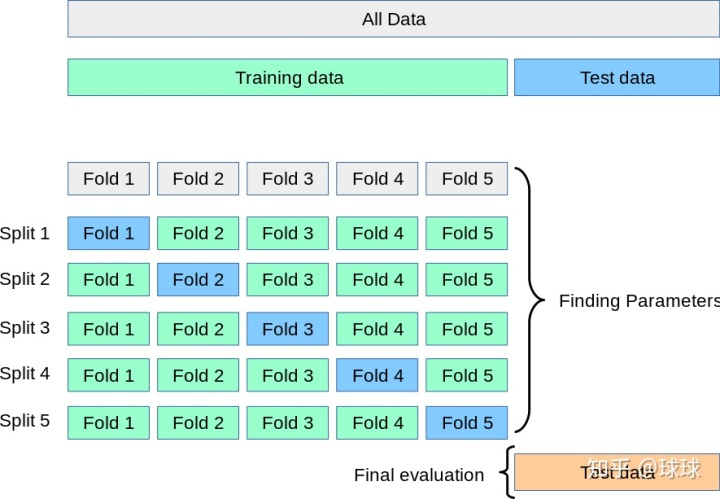图1cross_val_score与cross_validate在最新的版本sklearn 0.21中cross_val_score与cross_validate被统一，cross_val_score仅仅为调用cross_validate返回字典的结果。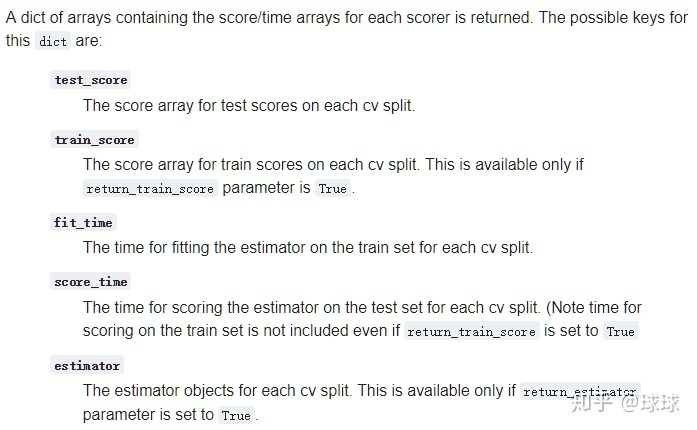cross_validate返回字典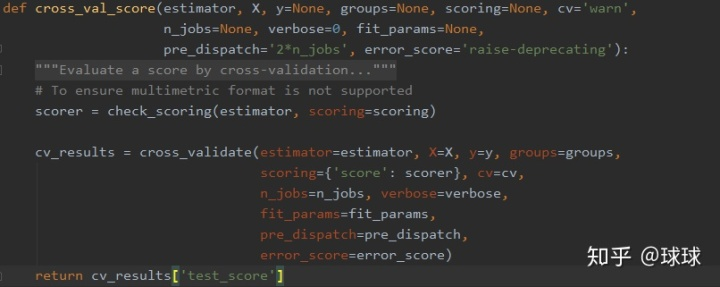图2cross_val_score，和cross_val_predictcross_val_score，和cross_val_predict 的分片方式相同，区别就是cross_val_predict的返回值不能直接用于计算得分评价！官网已经注明：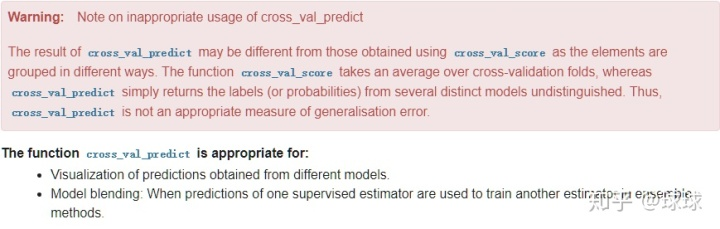意思是说，cross_val_predict返回的预测y值，是由分片的test y组合起来的，而这样y值的各个部分来源于不同的输入的学习器。查看源代码可以看到：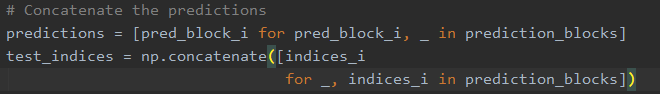把这些test y放在一起，看看预测值没问题，放在一起，评价得分，不合适！为什么呢？对比cross_val_score，我们发现道理很简单。当我们使用cross_val_predict计算得分时候，将采用与cross_val_score不同的计算策略，即：cross_val_score为先分片计算得分，后平均：score = np.mean(cross_val_score(estimator, data_x, y, cv=5))cross_val_predict为所有统一计算：predict_y = cross_val_predict(estimator, data_x, y, cv=5)
score = r2_score(true_y,predict_y)cross_val_score分片计算后平均的这种方式，可以认为是不同模型的平均结果，cross_val_predict计算得分没有道理可言。而且，这两种计算结果在数据量小的时候差别很大，所以遵循官网警告，谢绝使用cross_val_predict计算得分。那我一定要使用cross_val_predict计算得分怎么办呢？也很简单，使用相同的fold，把y划分计算再平均就好了.cv = KFold(n_splits=5, shuffle=False, random_state=0)
predict_y = cross_val_predict(estimator, data_x, y, cv=cv)

cv = KFold(n_splits=5, shuffle=False, random_state=0)  # 这是个generator,用完就没了,所以再来一遍
test_index = [i for i in cv.split(data_x)]
y_true_all = [y[_] for _ in test_index]
y_predict_all = [predict_y[_] for _ in test_index]

score = np.mean([r2_score(i, j) for i, j in zip(y_true_all, y_predict_all)])这样结果是和cross_val_score完全统一的，等价于cross_val_score。-----------------------------------------------------------------------------------------------ps : 重点来了，为什么要写这个短文，因为这个狗东西：r2_score注意事项sklearn.metrics.r2_score(y_true,y_pred,sample_weight=None)它用来衡量两列数值之间的趋势近似程度，如果按照感性的理解y_true,y_pred的位置应该是可以相互替换的，而且它的好朋友Pearsonr 系数的两个输入也是可以相互取代的。所以我就没有在乎输入顺序的问题，反正放进去就好了，从来没有关注过谁在前面。并且顺序弄反后，它不会报错，计算得分也看起来像那么回事，也在0-1之间。但是它不行！顺序不同，计算值得分是不同的！因为这个脑残的感性理解，我在发现代码结果与想象不同的时候，一度把矛头指向了GridSearch，cross_validate，cross_val_predict，cross_val_score。在查看一些stackoverflow上骗孩子(我)的解说后，怀疑这是cross_val_predict的切片方式和cross_val_score不同。然后从我自己的代码，一步一步debug到GridSearch, 到cross_val_predict，cross_val_score......展开全文• 在构建模型时，调参是极为重要的一个步骤，因为只有选择最佳的参数才能构建一个最优的模型。但是应该如何确定参数的值呢？所以这里记录一下选择参数的方法，以便后期复习以及分享。...sklearn 的 cross_val_sc...
在构建模型时，调参是极为重要的一个步骤，因为只有选择最佳的参数才能构建一个最优的模型。但是应该如何确定参数的值呢？所以这里记录一下选择参数的方法，以便后期复习以及分享。

（除了贝叶斯优化等方法）其它简单的验证有两种方法：1、通过经常使用某个模型的经验和高超的数学知识。2、通过交叉验证的方法，逐个来验证。

很显然我是属于后者所以我需要在这里记录一下

sklearn 的 cross_val_score：

我使用是cross_val_score方法，在sklearn中可以使用这个方法。交叉验证的原理不好表述下面随手画了一个图：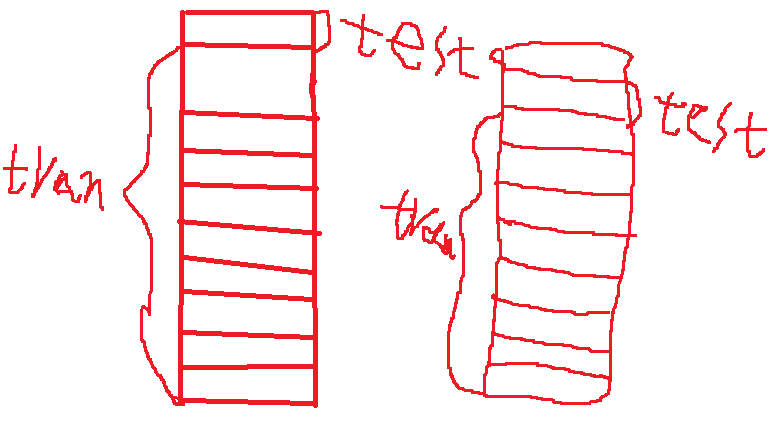（我都没见过这么丑的图）简单说下，比如上面，我们将数据集分为10折，做一次交叉验证，实际上它是计算了十次，将每一折都当做一次测试集，其余九折当做训练集，这样循环十次。通过传入的模型，训练十次，最后将十次结果求平均值。将每个数据集都算一次

交叉验证优点：

1：交叉验证用于评估模型的预测性能，尤其是训练好的模型在新数据上的表现，可以在一定程度上减小过拟合。
2：还可以从有限的数据中获取尽可能多的有效信息。

我们如何利用它来选择参数呢？

我们可以给它加上循环，通过循环不断的改变参数，再利用交叉验证来评估不同参数模型的能力。最终选择能力最优的模型。

下面通过一个简单的实例来说明：（iris鸢尾花）

from sklearn import datasets	#自带数据集
from sklearn.model_selection import train_test_split,cross_val_score	#划分数据 交叉验证
from sklearn.neighbors import KNeighborsClassifier  #一个简单的模型，只有K一个参数，类似K-means
import matplotlib.pyplot as plt
X = iris.data 			#这是数据
y = iris.target 		#这是每个数据所对应的标签
train_X,test_X,train_y,test_y = train_test_split(X,y,test_size=1/3,random_state=3)	#这里划分数据以1/3的来划分 训练集训练结果 测试集测试结果
k_range = range(1,31)
cv_scores = []		#用来放每个模型的结果值
for n in k_range:
knn = KNeighborsClassifier(n)   #knn模型，这里一个超参数可以做预测，当多个超参数时需要使用另一种方法GridSearchCV
scores = cross_val_score(knn,train_X,train_y,cv=10,scoring='accuracy')  #cv：选择每次测试折数  accuracy：评价指标是准确度,可以省略使用默认值，具体使用参考下面。
cv_scores.append(scores.mean())
plt.plot(k_range,cv_scores)
plt.xlabel('K')
plt.ylabel('Accuracy')		#通过图像选择最好的参数
plt.show()
best_knn = KNeighborsClassifier(n_neighbors=3)	# 选择最优的K=3传入模型
best_knn.fit(train_X,train_y)			#训练模型
print(best_knn.score(test_X,test_y))	#看看评分

最后得分0.94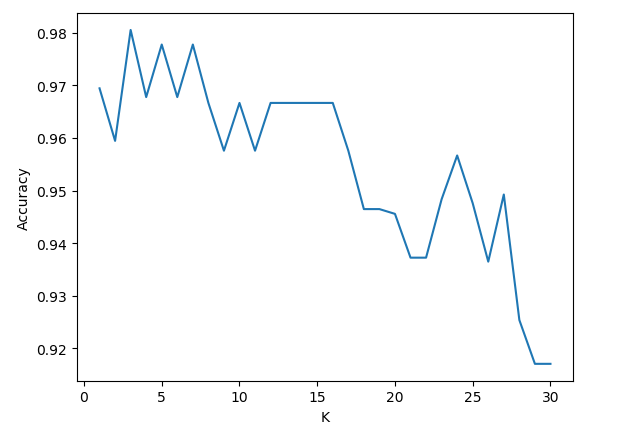关于 cross_val_score 的 scoring 参数的选择，通过查看官方文档后可以发现相关指标的选择可以在这里找到：文档。

这应该是比较简单的一个例子了，上面的注释也比较清楚，如果我表达不清楚可以问我。

以上都是个人理解，如果有问题还望指出。


展开全文sklearn
• ## cross_val_score的 scoring参数值解析

万次阅读 多人点赞 2018-09-24 20:56:07
一般我们在模型训练过程中，...sklearn.model_selection 的 cross_val_score 方法来计算模型的得分 scores = cross_val_score(clf, iris.data, iris.target, cv=5,scoring='accuracy') 我们看到这里有个参数 sc...
一般我们在模型训练过程中，会采用K折交叉验证的方法来验证模型的表现，从而进行调参，一般我们会用到

sklearn.model_selection 的 cross_val_score 方法来计算模型的得分

scores = cross_val_score(clf, iris.data, iris.target, cv=5,scoring='accuracy')

我们看到这里有个参数 scoring 参数，去scikit-learn官网了解之后发现这里的 scoring参数是默认为 None 的

sklearn.model_selection.cross_val_score(estimator, X, y=None, groups=None, scoring=None, cv=None, n_jobs=1, verbose=0, fit_params=None, pre_dispatch=‘2*n_jobs’)

scoring 参数可以有下面这些选择 具体参见 scoring-parameter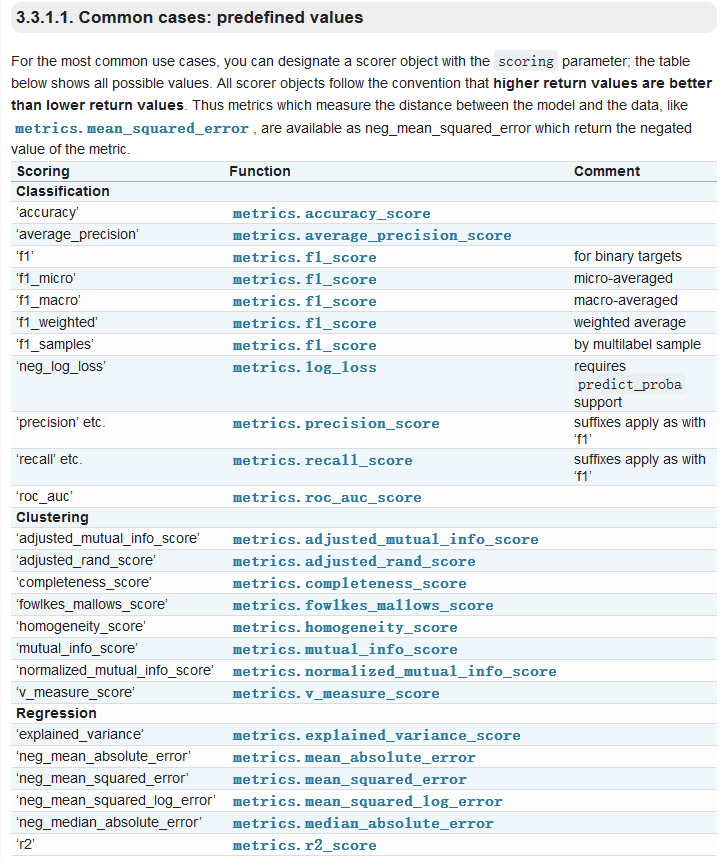这里文档对分类、聚类和回归三种问题下可以使用的参数进行了说明

分类（classification）问题中

常用的是 ‘precision’ 和 ’recall‘ 和 ’f1，三者的关系可以用下图来表示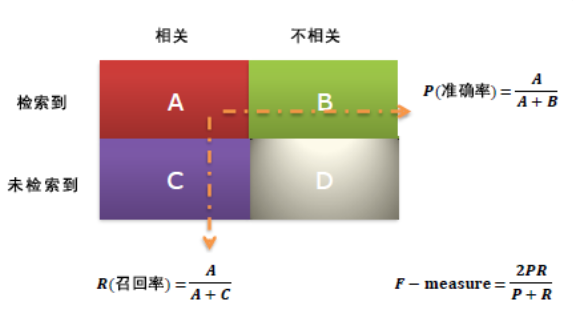假设这是一个二元分类的问题

准确率（precision）也就是被分类器检测到的数据中 分类正确的部分

召回率（recall）就是 正类中被分类正确的部分

而F1值就是 准确率和召回率的调和平均数

在实际应用中，如果是做搜索类的问题，那就是在保证召回率的情况下提升准确率

在做垃圾邮件检测之类的问题，就是要保证准确率的情况下提升召回率

具体也就是遇到具体问题看两者的权衡

如果两者都要求高，那就需要保证较高的F1 score

回归类（Regression）问题中

比较常用的是 'neg_mean_squared_error‘ 也就是 均方差回归损失

该统计参数是预测数据和原始数据对应点误差的平方和的均值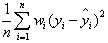公式长这样，了解下就ok了

以上属于个人的一点理解，不足之处大家可以随意吐槽


展开全文交叉验证 机器学习
• ## cross_val_score的参数

千次阅读 2018-12-03 16:04:21
https://scikit-learn.org/0.17/modules/generated/sklearn.cross_validation.cross_val_score.html 官网解释机器学习
• scikit-learn中的cross_val_score函数可以通过交叉验证评估分数，非常方便，但是使用过程中发现一个问题，就是在cross_val_score的文档中对scoring的参数并没有说明清楚。原始文档如下：文档中对scoring参数是这样...
• sklearn 中的cross_val_score函数可以用来进行交叉验证，因此十分常用，这里介绍这个函数的参数含义。 （本文内容来源于：https://blog.csdn.net/Asher117/article/details/87617702） sklearn.cross_validation....
• 在使用sklearn.model_selection的cross_val_score实现交叉验证时，我们也希望在fit时加入一些控制参数（比如sample_weight, eval_set, eval_metric, early_stopping_rounds等），以提高训练效率。 具体的实现方法...机器学习
• sklearn 中的cross_val_score函数可以用来进行交叉验证，因此十分常用，这里介绍这个函数的参数含义。 sklearn.cross_validation.cross_val_score(estimator, X, y=None, scoring=None, cv=None, n_jobs=1, ...Python sklearn
• 一、cross_val_score函数功能及参数释义 验证模型在某个训练集上的稳定性，输出cv=k个预测精度。 sklearn.cross_validation.cross_val_score( estimator, X, y=None, scoring=None, cv=None, n_jobs=1, verbose=0, ...交叉验证 sklearn
• 在使用交叉验证的过程中，我们...如果我们想要指定其他的评分方法就要使用到cross_val_score的scoring参数,图中将clf这个评估器的评分方法换成了f1_macro方法。 文档中还提到，当cv参数是整数或none时，且评估器是分.
• 1、sklearn.model_selection.cross_val_score(estimator,X,y=None,groups=None,scoring=None,cv=None,n_jobs=None,verbose=0,fit_params=None,pre_dispatch='2*n_jobs',error_score=nan) （1）cv参数定义交叉验证...机器学习
• scikit-learn中的cross_val_score函数可以通过交叉验证评估分数，非常方便，但是使用过程中发现一个问题，就是在cross_val_score的文档中对scoring的参数并没有说明清楚。其实有另一个文档进行了说明：3.3. Metrics ...
• 在构建模型时，调参是极为重要的一个...我使用是cross_val_score方法，在sklearn中可以使用这个方法。交叉验证的原理不好表述下面随手画了一个图： （我都没见过这么丑的图）简单说下，比如上面，我们将数据集分为10折
• sklearn中的cross_val_score()函数 sklearn.model_selection.cross_val_score(estimator, X, y=None, scoring=None, cv=None, n_jobs=1, verbose=0, fit_params=None, pre_dispatch=‘2*n_jobs’) 参数 ...机器学习
• 允许在scikit的cross_val_score使用多个度量功能的cross_val_score 。 正如已经讨论过的那样，Python的SciKit包含了用于计算估计量评估指标的强大功能（使用cross_val_score ），但在为同一分类器计算多个指标而不...
• 在构建模型时，调参是极为重要的一个步骤，因为只有选择最佳的参数才能构建一个最优的模型。但是应该如何确定参数的值呢？...很显然我是属于后者所以我需要在这里记录一下sklearn 的 cross_val_score：我使...
• from sklearn.model_selection import train_test_split,cross_val_score #划分数据 交叉验证 from sklearn.neighbors import KNeighborsClassifier #一个简单的模型，只有K一个参数，类似K-means impor...
• sklearn.cross_validation.cross_val_score(estimator, X, y=None, scoring=None, cv=None, n_jobs=1, verbose=0, fit_params=None, pre_dispatch=‘2*n_jobs’) 参数 estimator：需要使用交叉验证的算法 X：样本...机器学习
• 二、cross_val_score(选出最优评分的模型) 三、GridSearchCV(暴力搜索选出最优参数) 四、小结 cross_val_score ： 一般用于获取每折的交叉验证的得分，然后根据这个得分为模型选择合适的超参数，通常需要编写...sklearn
• 参考文章：...默认为None 其他选项如下： 1.accuracy:返回的值是正确率，等同于下面的方式。 from sklearn.metrics import accuracy_score y_pred=[0,2,1,3] ...
• 我在写我的问题的过程中解决了这个问题，所以在这里：cross_val_score的默认行为是使用KFold或StratifiedKFold来定义折叠。 默认情况下，两者都有参数shuffle=False ，因此不会从数据中随机抽取折叠：import numpy ...
• cross_val_score用来做交叉验证，里面的一个参数cv的选择比较悬疑，有时会用cv=n，有时又用cv=KFold(n_splits=n)。 查来查去，在《machine learning》终于找到了一个说法： When an integer is passed to ...
• 在构建模型时，调参是极为重要的一个步骤，因为只有选择最佳的参数才能构建一个最优的模型。但是应该如何确定参数的值呢？...很显然我是属于后者所以我需要在这里记录一下sklearn 的 cross_val_score：我使...